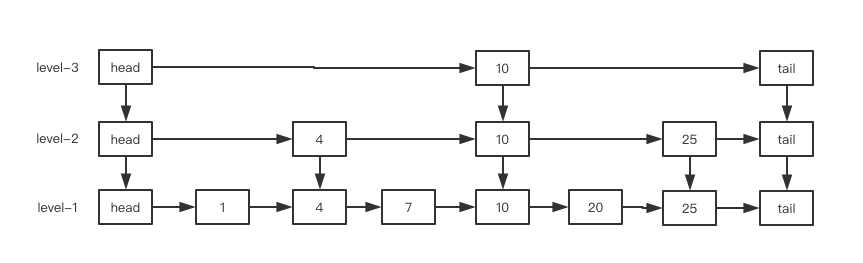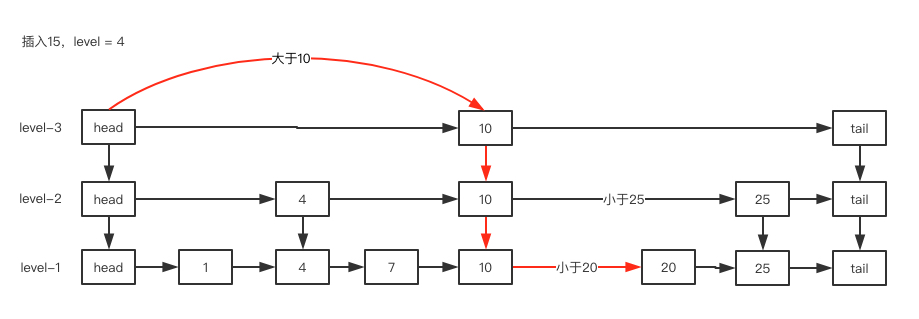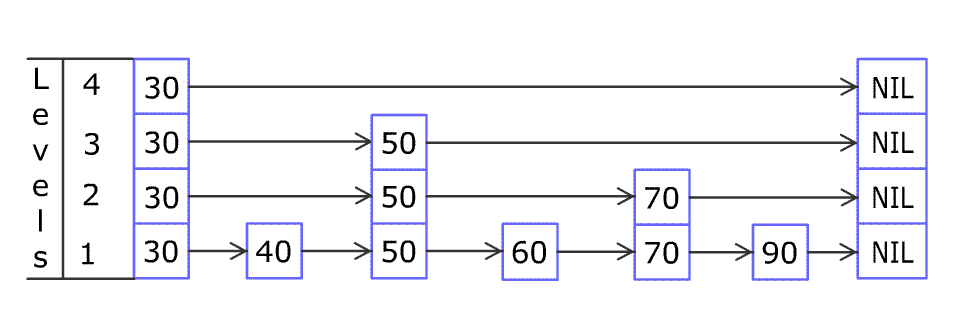# Java并发（9）- 从同步容器到并发容器

## 同步容器Vector和HashTable

//Vector
public synchronized int size() {};
public synchronized E get(int index) {};

//HashTable
public synchronized V put(K key, V value) {};
public synchronized V remove(Object key) {};


public static void deleteVector(){
int index = vectors.size() - 1;
vectors.remove(index);
}


public static void deleteVector(){
synchronized (vectors) {
int index = vectors.size() - 1;
vectors.remove(index);
}
}


public static void foreachVector(){
synchronized (vectors) {
for (int i = 0; i < vectors.size(); i++) {
System.out.println(vectors.get(i).toString());
}
}
}


## 并发容器CopyOnWrite

CopyOnWrite--写时复制容器是一种常用的并发容器，它通过多线程下读写分离来达到提高并发性能的目的，和前面我们讲解StampedLock时所用的解决方案类似：任何时候都可以进行读操作，写操作则需要加锁。不同的是，在CopyOnWrite中，对容器的修改操作加锁后，通过copy一个新的容器来进行修改，修改完毕后将容器替换为新的容器即可。

JDK中提供的并发容器包括CopyOnWriteArrayList和CopyOnWriteArraySet，下面通过CopyOnWriteArrayList的部分源码来理解这种思想：

//添加元素
//独占锁
final ReentrantLock lock = this.lock;
lock.lock();
try {
Object[] elements = getArray();
int len = elements.length;
//复制一个新的数组newElements
Object[] newElements = Arrays.copyOf(elements, len + 1);
newElements[len] = e;
//修改后指向新的数组
setArray(newElements);
return true;
} finally {
lock.unlock();
}
}

public E get(int index) {
//未加锁，直接获取
return get(getArray(), index);
}


CopyOnWrite容器虽然在多线程下使用是安全的，相比较Vector也大大提高了读写的性能，但它也有自身的问题。

## 并发容器ConcurrentHashMap

ConcurrentHashMap容器相较于CopyOnWrite容器在并发加锁粒度上有了更大一步的优化，它通过修改对单个hash桶元素加锁的达到了更细粒度的并发控制。在了解ConcurrentHashMap容器之前，推荐大家先阅读我之前对HashMap源码分析的文章--Java集合（5）一 HashMap与HashSet，因为在底层数据结构上，ConcurrentHashMap和HashMap都使用了数组+链表+红黑树的方式，只是在HashMap的基础上添加了并发相关的一些控制，所以这里只对ConcurrentHashMap中并发相关代码做一些分析。final V putVal(K key, V value, boolean onlyIfAbsent) {
if (key == null || value == null) throw new NullPointerException();
int binCount = 0;
//循环插入元素，避免并发插入失败
for (Node<K,V>[] tab = table;;) {
Node<K,V> f; int n, i, fh;
if (tab == null || (n = tab.length) == 0)
tab = initTable();
else if ((f = tabAt(tab, i = (n - 1) & hash)) == null) {
//如果当前桶无元素，则通过cas操作插入新节点
if (casTabAt(tab, i, null,
new Node<K,V>(hash, key, value, null)))
break;
}
//如果当前桶正在扩容，则协助扩容
else if ((fh = f.hash) == MOVED)
tab = helpTransfer(tab, f);
else {
V oldVal = null;
//hash冲突时锁住当前需要添加节点的头元素，可能是链表头节点或者红黑树的根节点
synchronized (f) {
if (tabAt(tab, i) == f) {
if (fh >= 0) {
binCount = 1;
for (Node<K,V> e = f;; ++binCount) {
K ek;
if (e.hash == hash &&
((ek = e.key) == key ||
(ek != null && key.equals(ek)))) {
oldVal = e.val;
if (!onlyIfAbsent)
e.val = value;
break;
}
Node<K,V> pred = e;
if ((e = e.next) == null) {
pred.next = new Node<K,V>(hash, key,
value, null);
break;
}
}
}
else if (f instanceof TreeBin) {
Node<K,V> p;
binCount = 2;
if ((p = ((TreeBin<K,V>)f).putTreeVal(hash, key,
value)) != null) {
oldVal = p.val;
if (!onlyIfAbsent)
p.val = value;
}
}
}
}
if (binCount != 0) {
if (binCount >= TREEIFY_THRESHOLD)
treeifyBin(tab, i);
if (oldVal != null)
return oldVal;
break;
}
}
}
return null;
}


• 如果当前桶对应的节点还没有元素插入，通过典型的无锁cas操作尝试插入新节点，减少加锁的概率，并发情况下如果插入不成功，很容易想到自旋，也就是for (Node<K,V>[] tab = table;;)
• 如果当前桶正在扩容，则协助扩容((fh = f.hash) == MOVED)。这里是一个重点，ConcurrentHashMap的扩容和HashMap不一样，它在多线程情况下或使用多个线程同时扩容，每个线程扩容指定的一部分hash桶，当前线程扩容完指定桶之后会继续获取下一个扩容任务，直到扩容全部完成。扩容的大小和HashMap一样，都是翻倍，这样可以有效减少移动的元素数量，也就是使用2的幂次方的原因，在HashMap中也一样。
• 在发生hash冲突时仅仅只锁住当前需要添加节点的头元素即可，可能是链表头节点或者红黑树的根节点，其他桶节点都不需要加锁，大大减小了锁粒度。

## 并发容器ConcurrentSkipListMap

java.util中对应的容器在java.util.concurrent包中基本都可以找到对应的并发容器：List和Set有对应的CopyOnWriteArrayList与CopyOnWriteArraySet，HashMap有对应的ConcurrentHashMap，但是有序的TreeMap或并没有对应的ConcurrentTreeMap。

ConcurrentSkipListMap内部使用跳表（SkipList）这种数据结构来实现，他的结构相对红黑树来说非常简单理解，实现起来也相对简单，而且在理论上它的查找、插入、删除时间复杂度都为log(n)。在并发上，ConcurrentSkipListMap采用无锁的CAS+自旋来控制。

int random_level()  {
K = 1;
while (random(0,1))
K++;

return K;
}private V doPut(K key, V value, boolean onlyIfAbsent) {
if (key == null)
throw new NullPointerException();
Comparator<? super K> cmp = comparator;
outer: for (;;) {
for (Node<K,V> b = findPredecessor(key, cmp), n = b.next;;) { //查找前继节点
if (n != null) { //查找到前继节点
Object v; int c;
Node<K,V> f = n.next; //获取后继节点的后继节点
if (n != b.next)  //发生竞争，两次节点获取不一致，并发导致
break;
if ((v = n.value) == null) {  // 节点已经被删除
n.helpDelete(b, f);
break;
}
if (b.value == null || v == n)
break;
if ((c = cpr(cmp, key, n.key)) > 0) { //进行下一轮查找，比当前key大
b = n;
n = f;
continue;
}
if (c == 0) { //相等时直接cas修改值
if (onlyIfAbsent || n.casValue(v, value)) {
@SuppressWarnings("unchecked") V vv = (V)v;
return vv;
}
break; // restart if lost race to replace value
}
// else c < 0; fall through
}

z = new Node<K,V>(key, value, n); //9. n.key > key > b.key
if (!b.casNext(n, z)) //cas修改值
break;         // restart if lost race to append to b
break outer;
}
}

if ((rnd & 0x80000001) == 0) { // test highest and lowest bits
int level = 1, max;
while (((rnd >>>= 1) & 1) != 0) // 获取跳表层级
++level;
Index<K,V> idx = null;
if (level <= (max = h.level)) { //如果获取的调表层级小于等于当前最大层级，则直接添加，并将它们组成一个上下的链表
for (int i = 1; i <= level; ++i)
idx = new Index<K,V>(z, idx, null);
}
else { // try to grow by one level //否则增加一层level，在这里体现为Index<K,V>数组
level = max + 1; // hold in array and later pick the one to use
@SuppressWarnings("unchecked")Index<K,V>[] idxs =
(Index<K,V>[])new Index<?,?>[level+1];
for (int i = 1; i <= level; ++i)
idxs[i] = idx = new Index<K,V>(z, idx, null);
for (;;) {
int oldLevel = h.level;
if (level <= oldLevel) // lost race to add level
break;
Node<K,V> oldbase = h.node;
for (int j = oldLevel+1; j <= level; ++j) //新添加的level层的具体数据
newh = new HeadIndex<K,V>(oldbase, newh, idxs[j], j);
h = newh;
idx = idxs[level = oldLevel];
break;
}
}
}
// 逐层插入数据过程
splice: for (int insertionLevel = level;;) {
int j = h.level;
for (Index<K,V> q = h, r = q.right, t = idx;;) {
if (q == null || t == null)
break splice;
if (r != null) {
Node<K,V> n = r.node;
// compare before deletion check avoids needing recheck
int c = cpr(cmp, key, n.key);
if (n.value == null) {
break;
r = q.right;
continue;
}
if (c > 0) {
q = r;
r = r.right;
continue;
}
}

if (j == insertionLevel) {
break; // restart
if (t.node.value == null) {
findNode(key);
break splice;
}
if (--insertionLevel == 0)
break splice;
}

if (--j >= insertionLevel && j < level)
t = t.down;
q = q.down;
r = q.right;
}
}
}
return null;
}


## 总结

JDK中提供了丰富的并发容器供我们使用，文章中介绍的也并不全面，重点是要通过了解各种并发容器的原理，明白他们各自独特的使用场景。这里简单做个总结：当并发读远多于修改的场景下需要使用List和Set时，可以考虑使用CopyOnWriteArrayList和CopyOnWriteArraySet；当需要并发使用<Key, Value>键值对存取数据时，可以使用ConcurrentHashMap；当要保证并发<Key, Value>键值对有序时可以使用ConcurrentSkipListMap。

posted @ 2018-10-17 07:34  knock_小新  阅读(4821)  评论(2编辑  收藏